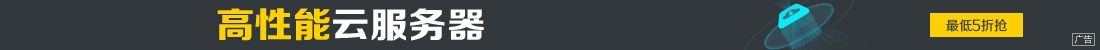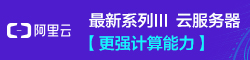# oracle 存储过程、函数和触发器用法实例详解

••```create [or replace] procedure 过程名(参数列表)
as
PLSQL子程序体;```
```--给指定员工涨工资
as
psal emp.sal%type;
begin
select sal into psal from emp where empno=empid;
update emp set sal = sal * 1.1 where empno=empid;
dbms_output.put_line(empid || '涨工资前' || psal || '涨工资后' || (psal * 1.1));
end;

```

```--方法一
begin
end;
--方法二

```

```CREATE [OR REPLACE] FUNCTION 函数名 (参数列表)
RETURN 函数值类型
AS
PLSQL子程序体;

```
```--查询指定员工的年收入
create function queryEmpSal(empid in number)
return number
as
psal emp.sal%type;
pcomm emp.comm%type;
begin
select sal,comm into psal,pcomm from emp where empno=empid;
return (psal*12) + nvl(pcomm,0);
end;

```

```declare
psal number;
begin
psal:=queryEmpSal(7369);
dbms_output.put_line(psal);
end;

```

```begin
dbms_output.put_line(queryEmpSal(7369));
end;

```

```--包定义
create [or replace] package 包名 as
[公有数据类型定义]
[公有游标声明]
[公有变量、常量声明]
[公有子程序声明]
end 包名;

```
```--包主体
create [or replace] package body 包名 as
[私有数据类型定义]
[私有变量、常量声明]
[私有子程序声明和定义]
[公有子程序定义]
begin
PL/SQL子程序体;
end 包名;

```
```--创建mypackage包
create or replace package mypackage as
procedure total(num1 in number, num2 in number, num3 out number);
end mypackage;

```
```--mypackage包体
create or replace package body mypackage as
--计算累加和的total过程
procedure total(num1 in number, num2 in number, num3 out number) as
tmp number := num1;
begin
if num2 < num1 then num3 := 0;
else num3 := tmp;
loop
exit when tmp > num2;
tmp := tmp + 1;
num3 := num3 + tmp;
end loop;
end if;
end total;

end mypackage;

```

(*注意：包定义和包体要分开创建)

```declare
num1 number;
begin
mypackage.total(1, 5, num1);
dbms_output.put_line(num1);
end;

```